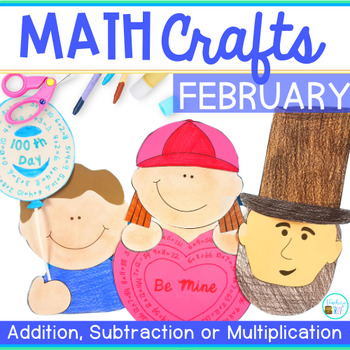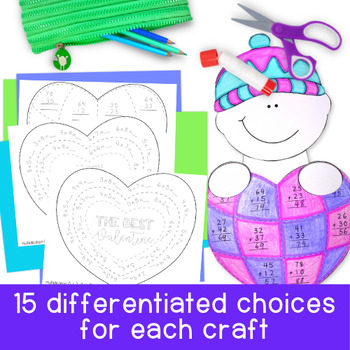Math Crafts | Valentines Day | 100th Day of School1st - 3rd
Subjects
Standards
Resource Type
Formats Included
• PDF
Pages
62 pages

Also included in

1. Math crafts are an engaging way for your students to practice their math skills. This huge bundle of monthly themed packs allows you to differentiate math activities so your students can work on their addition, subtraction and multiplication facts or consolidate adding and subtracting two or three d
\$25.00
\$49.50
Save \$24.50

Description

Math craftivities are an engaging way for your students to practice their math skills.

This February themed pack allows you to differentiate math activities so your students can work on their addition, subtraction and multiplication facts or consolidate adding and subtracting two or three digit numbers. Whichever concept they need more practice with.

Your class will be so motivated to complete these fun math craftivities they won’t even realize they’re doing work!

This pack contains 3 math crafts for February: the 100th day of school, President’s day and Valentine’s Day.

Each craft has 15 different math options:

• Subtraction to 10
• Subtraction to 20
• 2 digit addition without regrouping
• 2 digit subtraction without regrouping
• 2 digit addition with and without regrouping
• 2 digit subtraction with and without regrouping
• 3 digit subtraction
• Multiplication facts – 0, 1, 2, 5, 10x
• Multiplication facts – 0, 1, 2, 3, 4, 5x
• Multiplication facts – 6, 7, 8, 9x
• Multiplication facts – 9, 10, 11, 12
• Multiplication facts – to 10x

Become a follower and take advantage of my Early Bird Specials, freebies and product launches. Look for the green star near the top of any page within my store and click it to become a follower. You will then receive customized email updates about this store.

Would you like to receive TPT credit to use on future purchases? Just go to your My Purchases page (you may need to login). Beside each purchase you'll see a Provide Feedback button. Simply click it and you will be taken to a page where you can give a quick rating and leave a short comment for the product. I value your feedback greatly as it helps me determine which products are most valuable for your classroom so I can create more for you.

Total Pages
62 pages
Not Included
Teaching Duration
N/A
Report this Resource to TpT
Reported resources will be reviewed by our team. Report this resource to let us know if this resource violates TpT’s content guidelines.

Standards

to see state-specific standards (only available in the US).
Fluently multiply and divide within 100, using strategies such as the relationship between multiplication and division (e.g., knowing that 8 × 5 = 40, one knows 40 ÷ 5 = 8) or properties of operations. By the end of Grade 3, know from memory all products of two one-digit numbers.
Fluently add and subtract within 20 using mental strategies. By end of Grade 2, know from memory all sums of two one-digit numbers.
Add and subtract within 20, demonstrating fluency for addition and subtraction within 10. Use strategies such as counting on; making ten (e.g., 8 + 6 = 8 + 2 + 4 = 10 + 4 = 14); decomposing a number leading to a ten (e.g., 13 - 4 = 13 - 3 - 1 = 10 - 1 = 9); using the relationship between addition and subtraction (e.g., knowing that 8 + 4 = 12, one knows 12 - 8 = 4); and creating equivalent but easier or known sums (e.g., adding 6 + 7 by creating the known equivalent 6 + 6 + 1 = 12 + 1 = 13).
Add and subtract within 1000, using concrete models or drawings and strategies based on place value, properties of operations, and/or the relationship between addition and subtraction; relate the strategy to a written method. Understand that in adding or subtracting three-digit numbers, one adds or subtracts hundreds and hundreds, tens and tens, ones and ones; and sometimes it is necessary to compose or decompose tens or hundreds.
Fluently add and subtract within 100 using strategies based on place value, properties of operations, and/or the relationship between addition and subtraction.